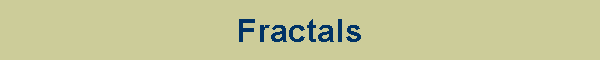Koch Snowflake      The Koch snowflake is a curve of infinite length with a dimension of 1.261859. It is constructed by taking an equilateral triangle, dividing each side into three equal parts, erasing the middle part and inserting an equilateral triangle into the middle of each side. The process is then repeated as many times as desired. The curve is named after Niels Fabian Helge von Koch (Sweden, 1870-1924) who developed the curve around 1905. Julia Set      The term "fractal" was coined by Benoit Mandelbrot in 1975. Fractals are non-regular geometric shapes that have the same degree of non-regularity at all scales. Fractals are self-similar whether you view them from close up or very far away.      The Mandelbrot Set is a fractal is produced by the formula zz+1=zn2 + c, where z and c are complex numbers, z0=0, and c is a variable point on the plane. The formula is iterated until |zn| ≥2.      The Julia Set, described by Gaston Julia in 1918, is also a fractal which is based on the same formula with c as the constant and z as the variable. There is a Julia Set for each point on the complex plane. The stamp shows a part of the pattern produced when c=0.2860 + 0.0115i.